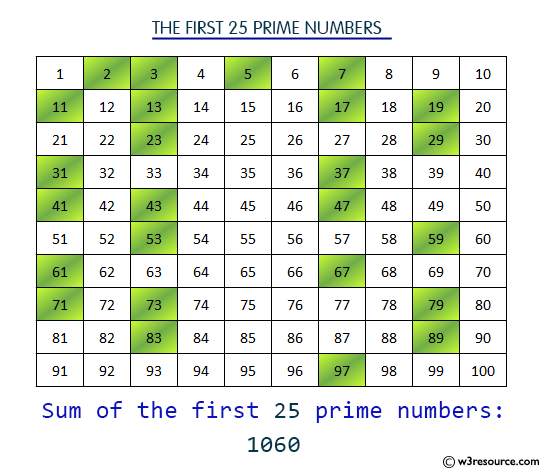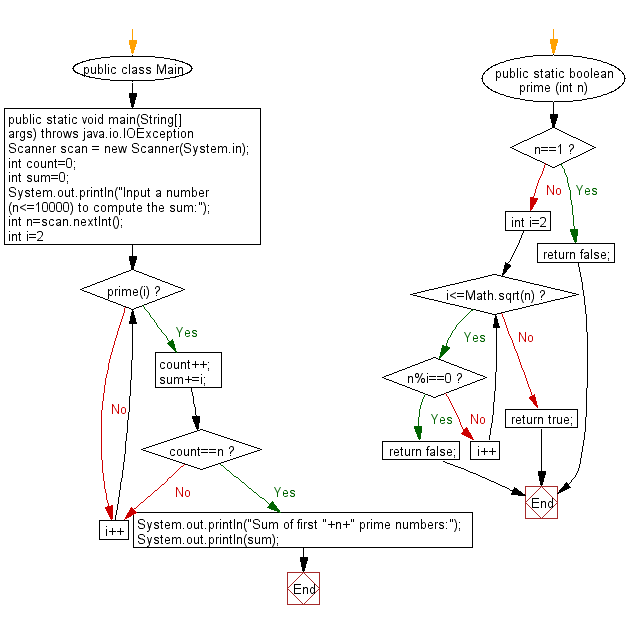# Java Exercises: Compute the sum of first n given prime numbers

## Java Basic: Exercise-232 with Solution

Write a Java program to compute the sum of first n given prime numbers.

Input:

n ( n ≤ 10000). Input 0 to exit the program.

Pictorial Presentation:Sample Solution:

Java Code:

``````import java.util.*;
public class Main {

public static void main(String[] args) throws java.io.IOException{
Scanner scan = new Scanner(System.in);
int count=0;
int sum=0;
System.out.println("Input a number (n<=10000) to compute the sum:");
int n=scan.nextInt();
for(int i=2;;i++){
if(prime(i)){
count++;
sum+=i;
if(count==n)break;
}
}
System.out.println("Sum of first "+n+" prime numbers:");
System.out.println(sum);
}
public static boolean prime (int n){
if(n==1)return false;
for(int i=2;i<=Math.sqrt(n);i++)
if(n%i==0)return false;
return true;
}
}
```
```

Sample Output:

```Input a number (n<=10000) to compute the sum:
100
Sum of first 100 prime numbers:
24133
```

Flowchart:Java Code Editor:

What is the difficulty level of this exercise?

﻿

## Java: Tips of the Day

Array vs ArrayLists:

The main difference between these two is that an Array is of fixed size so once you have created an Array you cannot change it but the ArrayList is not of fixed size. You can create instances of ArrayLists without specifying its size. So if you create such instances of an ArrayList without specifying its size Java will create an instance of an ArrayList of default size.

Once an ArrayList is full it re-sizes itself. In fact, an ArrayList is internally supported by an array. So when an ArrayList is resized it will slow down its performance a bit as the contents of the old Array must be copied to a new Array.

At the same time, it's compulsory to specify the size of an Array directly or indirectly while creating it. And also Arrays can store both primitives and objects while ArrayLists only can store objects.

Ref: https://bit.ly/3o8L2KH